This can take objects of type Raster*, SpatialPoints*, SpatialPolygons*, and any combination of those. These can be provided as individual objects, or a named list. If a named list, the names either represent a different original object in the calling environment and that will be used, or if the names don't exist in the calling environment, then they will be copied to .quickPlotEnv for reuse later. It can also handle ggplot2 objects or base::histogram objects created via call to exHist <- hist(1:10, plot = FALSE). It can also take arguments as if it were a call to plot. In this latter case, the user should be explicit about naming the plot area using addTo. Customization of the ggplot2 elements can be done as a normal ggplot2 plot, then added with Plot(ggplotObject).

Plot(..., new = FALSE, addTo = NULL, gp = gpar(), gpText = gpar(),
gpAxis = gpar(), axes = FALSE, speedup = 1, size = 5,
cols = NULL, col = NULL, zoomExtent = NULL, visualSqueeze = NULL,
legend = TRUE, legendRange = NULL, legendText = NULL, pch = 19,
title = NULL, na.color = "#FFFFFF00", zero.color = NULL,
length = NULL, arr = NULL, plotFn = "plot")

# S4 method for ANY
Plot(..., new = FALSE, addTo = NULL, gp = gpar(),
gpText = gpar(), gpAxis = gpar(), axes = FALSE, speedup = 1,
size = 5, cols = NULL, col = NULL, zoomExtent = NULL,
visualSqueeze = NULL, legend = TRUE, legendRange = NULL,
legendText = NULL, pch = 19, title = NULL,
na.color = "#FFFFFF00", zero.color = NULL, length = NULL,
arr = NULL, plotFn = "plot")

rePlot(toDev = dev.cur(), fromDev = dev.cur(), clearFirst = TRUE,
...)

## Arguments

... A combination of spatialObjects or non-spatial objects. For many object classes, there are specific Plot methods. Where there are no specific ones, the base plotting will be used internally. This means that for objects with no specific Plot methods, many arguments, such as addTo, will not work. See details. Logical. If TRUE, then the previous named plot area is wiped and a new one made; if FALSE, then the ... plots will be added to the current device, adding or rearranging the plot layout as necessary. Default is FALSE. This currently works best if there is only one object being plotted in a given Plot call. However, it is possible to pass a list of logicals to this, matching the length of the ... objects. Use clearPlot to clear the whole plotting device. Character vector, with same length as .... This is for overplotting, when the overplot is not to occur on the plot with the same name, such as plotting a SpatialPoints* object on a RasterLayer. A gpar object, created by gpar function, to change plotting parameters (see grid package). A gpar object for the title text. Default gpar(col = "black"). A gpar object for the axes. Default gpar(col = "black"). Logical or "L", representing the left and bottom axes, over all plots. Numeric. The factor by which the number of pixels is divided by to plot rasters. See Details. Numeric. The size, in points, for SpatialPoints symbols, if using a scalable symbol. (also col) Character vector or list of character vectors of colours. See details. (also cols) Alternative to cols to be consistent with plot. cols takes precedence, if both are provided. An Extent object. Supplying a single extent that is smaller than the rasters will call a crop statement before plotting. Defaults to NULL. This occurs after any downsampling of rasters, so it may produce very pixelated maps. Numeric. The proportion of the white space to be used for plots. Default is 0.75. Logical indicating whether a legend should be drawn. Default is TRUE. Numeric vector giving values that, representing the lower and upper bounds of a legend (i.e., 1:10 or c(1,10) will give same result) that will override the data bounds contained within the grobToPlot. Character vector of legend value labels. Defaults to NULL, which results in a pretty numeric representation. If Raster* has a Raster Attribute Table (rat; see raster package), this will be used by default. Currently, only a single vector is accepted. The length of this must match the length of the legend, so this is mostly useful for discrete-valued rasters. see ?par. Logical or character string. If logical, it indicates whether to print the object name as the title above the plot. If a character string, it will print this above the plot. NOTE: the object name is used with addTo, not the title. Default NULL, which means print the object name as title, if no other already exists on the plot, in which case, keep the previous title. Character string indicating the color for NA values. Default transparent. Character string indicating the color for zero values, when zero is the minimum value, otherwise, zero is treated as any other color. Default transparent. Numeric. Optional length, in inches, of the arrow head. A vector of length 2 indicating a desired arrangement of plot areas indicating number of rows, number of columns. Default NULL, meaning let Plot function do it automatically. An optional function name to do the plotting internally, e.g., "barplot" to get a barplot() call. Default "plot". Numeric. Which device should the new rePlot be plotted to. Default is current device. Numeric. Which device should the replot information be taken from. Default is current device Logical. Should clearPlot be run before replotting. Default TRUE.

## Value

Invisibly returns the .quickPlot class object. If this is assigned to an object, say obj, then this can be plotted again with Plot(obj). This object is also stored in the locked .quickPlotEnv, so can simply be replotted with rePlot() or on a new device with rePlot(n), where n is the new device number.

## Details

NOTE: Plot uses the grid package; therefore, it is NOT compatible with base R graphics. Also, because it does not by default wipe the plotting device before plotting, a call to clearPlot is helpful to resolve many errors. Careful use of the other device tools, such as dev.off() and dev.list() might also clear problems that may arise.

If new = TRUE, a new plot will be generated, but only in the figure region that has the same name as the object being plotted. This is different than calling clearPlot(); Plot(Object), i.e,. directly before creating a new Plot. clearPlot() will clear the entire plotting device. When new = FALSE, any plot that already exists will be overplotted, while plots that have not already been plotted will be added. This function rearranges the plotting device to maximize the size of all the plots, minimizing white space. If using the RStudio IDE, it is recommended to make and use a new device with dev(), because the built in device is not made for rapid redrawing. The function is based on the grid package.

Each panel in the multipanel plot must have a name. This name is used to overplot, rearrange the plots, or overlay using addTo when necessary. If the ... are named spatialObjects, then Plot will use these names. However, this name will not persist when there is a future call to Plot that forces a rearrangement of the plots. A more stable way is to use the object names directly, and any layer names (in the case of RasterLayer or RasterStack objects). If plotting a RasterLayer and the layer name is "layer" or the same as the object name, then, for simplicity, only the object name will be used. In other words, only enough information is used to uniquely identify the plot.

Because of modularity, Plot must have access to the original objects that were plotted. These objects will be used if a subsequent Plot event forces a rearrangement of the Plot device. Rather than saving all the plot information (including the data) at each Plot call (this is generally too much data to constantly make copies), the function saves a pointer to the original R object. If the plot needs to be rearranged because of a future addition, then Plot will search for that original object that created the first plots, and replot them. This has several consequences. First, that object must still exist and in the same environment. Second, if that object has changed between the first time it is plot and any subsequent time it is replotted (via a forced rearrangement), then it will take the object *as it exists*, not as it existed. Third, if passing a named list of objects, Plot will either create a link to objects with those names in the calling environment (e.g., .GlobalEnv) or, if they do not exist, then Plot will make a copy in the hidden .quickPlotEnv for later reuse.

cols is a vector of colours that can be understood directly, or by colorRampePalette, such as c("orange", "blue"), will give a colour range from orange to blue, interploated. If a list, it will be used, in order, for each item to be plotted. It will be recycled if it is shorter than the objects to be plotted. Note that when this approach to setting colours is used, any overplotting will revert to the colortable slot of the object, or the default for rasters, which is terrain.color()

cols can also accept RColorBrewer colors by keyword if it is character vector of length 1. i.e., this cannot be used to set many objects by keyword in the same Plot call. Default terrain.color(). See Details.

Some coloring will be automatic. If the object being plotted is a Raster, then this will take the colorTable slot (can be changed via setColors() or other ways). If this is a SpatialPointsDataFrame, this function will use a column called colors and apply these to the symbols.

Silently, one hidden object is made, .quickPlot in the .quickPlotEnv environment, which is used for arranging plots in the device window, and identifying the objects to be replotted if rearranging is required, subsequent to a new = FALSE additional plot.

This function is optimized to allow modular Plotting. This means that several behaviours will appear unusual. For instance, if a first call to Plot is made, the legend will reflect the current color scheme. If a second or subsequent call to Plot is made with the same object but with different colours (e.g., with cols), the legend will not update. This behaviour is made with the decision that the original layer takes precedence and all subsequent plots to that same frame are overplots only.

speedup is not a precise number because it is faster to plot an non-resampled raster if the new resampling is close to the original number of pixels. At the moment, for rasters, this is set to 1/3 of the original pixels. In other words, speedup will not do anything if the factor for speeding up is not high enough (i.e., >3). If no sub-sampling is desired, use a speedup value less than 0.1.

These gp* parameters will specify plot parameters that are available with gpar(). gp will adjust plot parameters, gpText will adjust title and legend text, gpAxis will adjust the axes. size adjusts point size in a SpatialPoints object. These will persist with the original Plot call for each individual object. Multiple entries can be used, but they must be named list elements and they must match the ... items to plot. This is true for a RasterStack also, i.e., the list of named elements must be the same length as the number of layers being plotted. The naming convention used is: RasterStackName$layerName, i.e, landscape$DEM.

clearPlot, gpar, raster, par, SpatialPolygons, grid.polyline, ggplot, dev

## Examples

library(sp)
library(raster)
library(RColorBrewer)

files <- dir(system.file("maps", package = "quickPlot"), full.names = TRUE, pattern = "tif")
maps <- lapply(files, raster)
names(maps) <- lapply(maps, names)

# put layers into a single stack for convenience
landscape <- stack(maps$DEM, maps$forestCover, maps$forestAge, maps$habitatQuality, maps$percentPine) # can change color palette setColors(landscape, n = 50) <- list(DEM = topo.colors(50), forestCover = brewer.pal(9, "Set1"), forestAge = brewer.pal("Blues", n = 8), habitatQuality = brewer.pal(9, "Spectral"), percentPine = brewer.pal("GnBu", n = 8)) # Make a new raster derived from a previous one; must give it a unique name habitatQuality2 <- landscape$habitatQuality ^ 0.3
names(habitatQuality2) <- "habitatQuality2"

# make a SpatialPoints object
caribou <- sp::SpatialPoints(coords = cbind(x = stats::runif(1e2, -50, 50),
y = stats::runif(1e2, -50, 50)))

# use factor raster to give legends as character strings
ras <- raster(extent(0, 3, 0, 4), vals = sample(1:4, size = 12, replace = TRUE), res = 1)

# needs to have a data.frame with ID as first column - see ?raster::ratify
levels(ras) <- data.frame(ID = 1:4, Name = paste0("Level", 1:4))
Plot(ras, new = TRUE)
# Arbitrary values for factors, including zero and not all levels represented in raster
levs <- c(0:5, 7:12)
ras <- raster(extent(0, 3, 0, 2), vals = c(1, 1, 3, 5, 8, 9), res = 1)
levels(ras) <- data.frame(ID = levs, Name = LETTERS[c(1:3, 8:16)])
Plot(ras, new = TRUE)
# Arbitrary values for factors, including zero and not all levels represented in raster
levs <- c(0:5, 7:23)
ras <- raster(extent(0, 3, 0, 2), vals = c(1, 1, 3, 5, 8, 9), res = 1)
levels(ras) <- data.frame(ID = levs, Name = LETTERS[1:23])
Plot(ras, new = TRUE)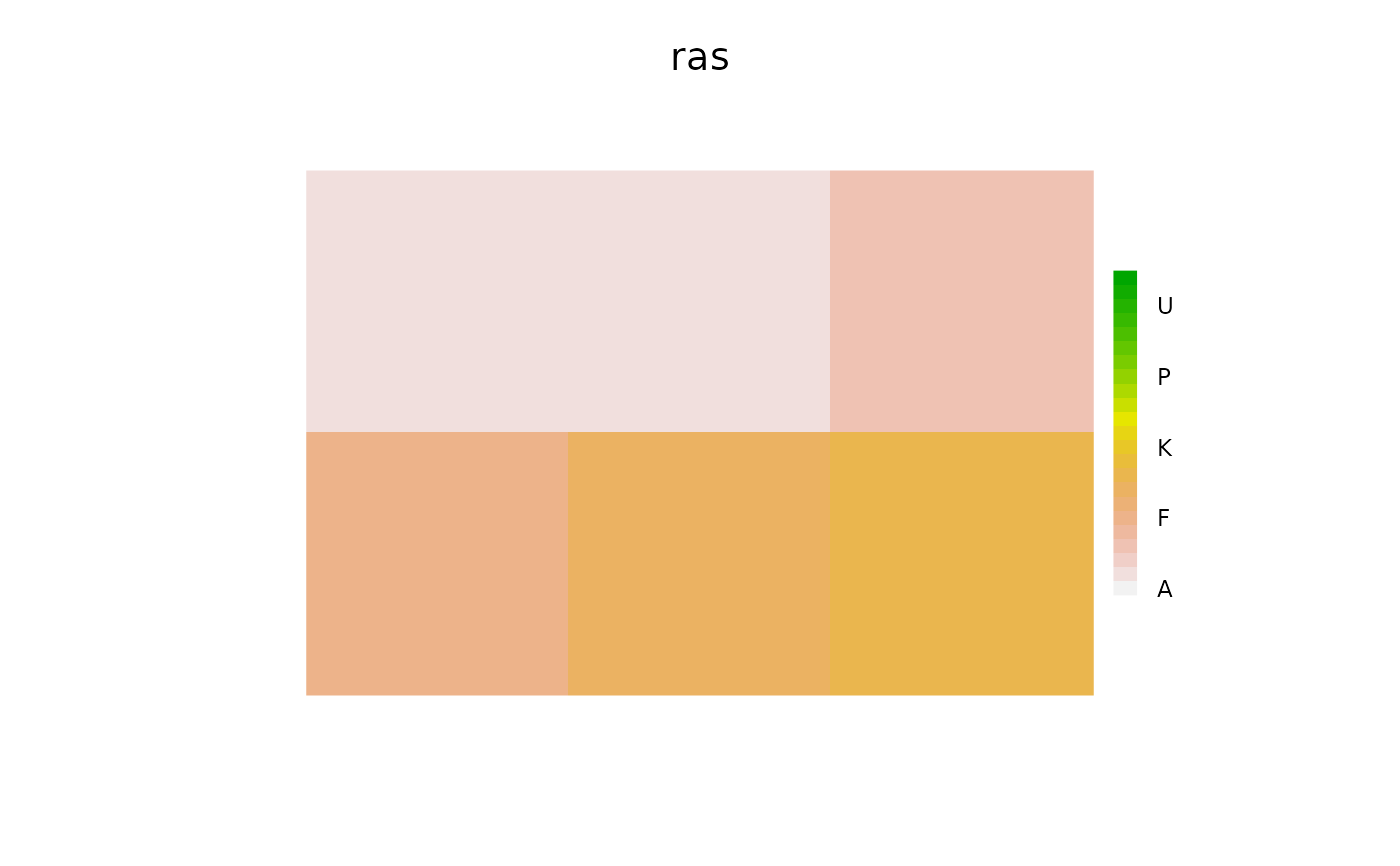# SpatialPolygons
sr1 <- sp::Polygon(cbind(c(2, 4, 4, 1, 2), c(2, 3, 5, 4, 2)) * 20 - 50)
sr2 <- sp::Polygon(cbind(c(5, 4, 2, 5), c(2, 3, 2, 2)) * 20 - 50)
srs1 <- sp::Polygons(list(sr1), "s1")
srs2 <- sp::Polygons(list(sr2), "s2")
spP <- sp::SpatialPolygons(list(srs1, srs2), 1:2)

clearPlot()Plot(ras)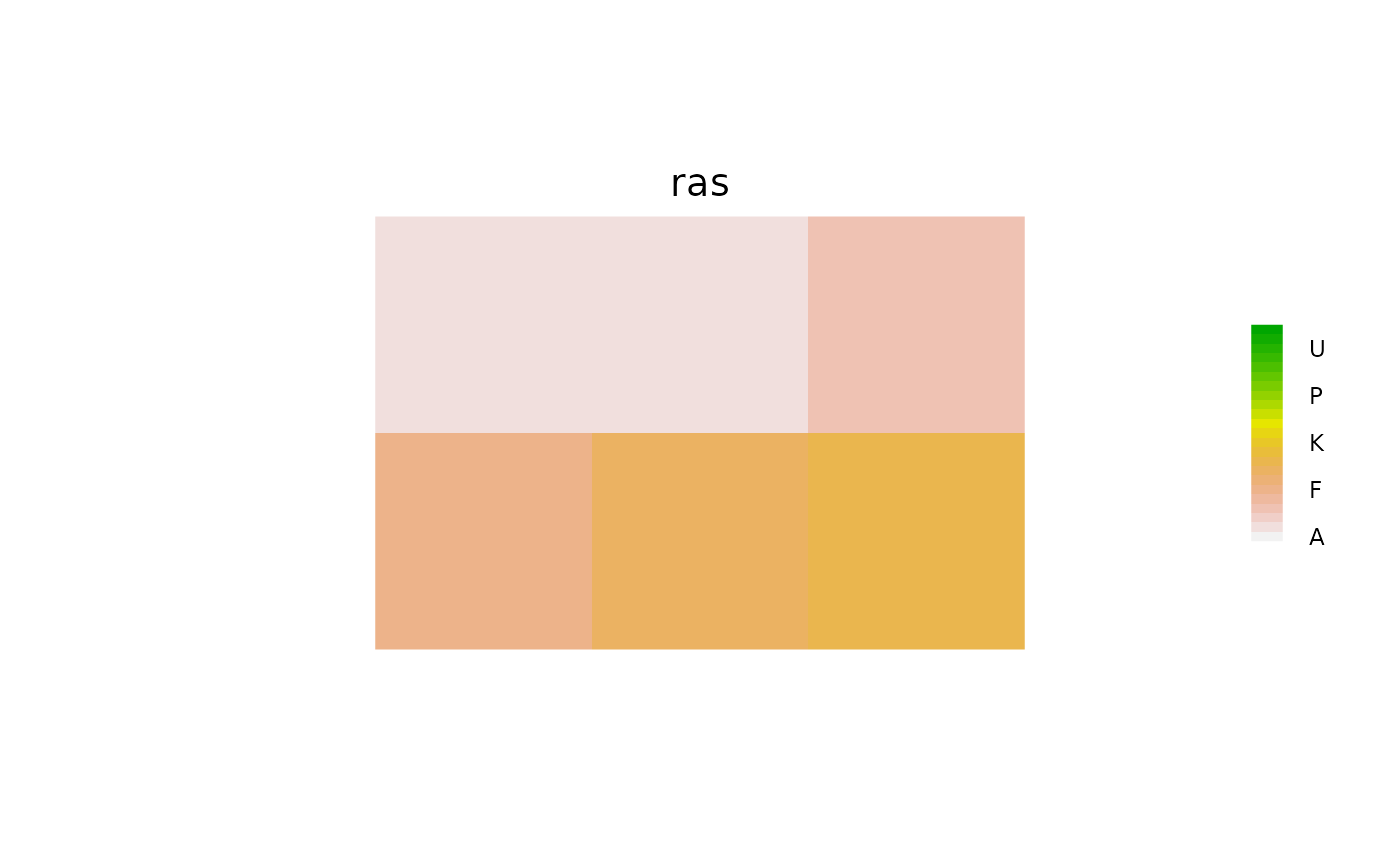clearPlot()Plot(landscape)
Plot(caribou, addTo = "landscape$forestAge", size = 4, axes = FALSE) # can add a plot to the plotting window Plot(caribou, new = FALSE)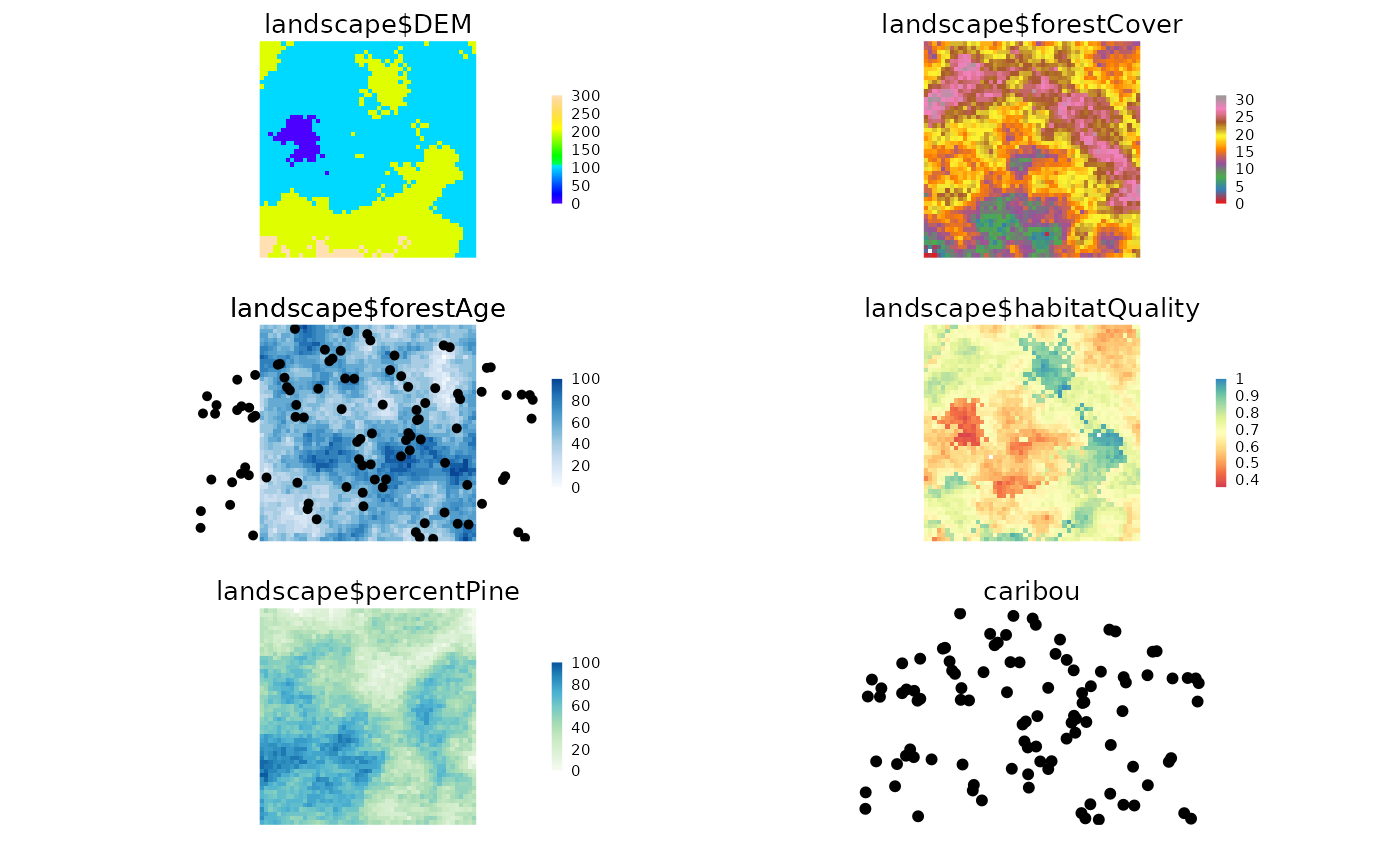# Can add two maps with same name, if one is in a stack; they are given # unique names based on object name Plot(landscape, caribou, maps$DEM)
Plot(landscape, caribou)Plot(habitatQuality2, new = FALSE)Plot(spP)Plot(spP, addTo = "landscape$forestCover", gp = gpar(lwd = 2)) # provide arrangement, NumRow, NumCol Plot(spP, arr = c(1, 4), new = TRUE)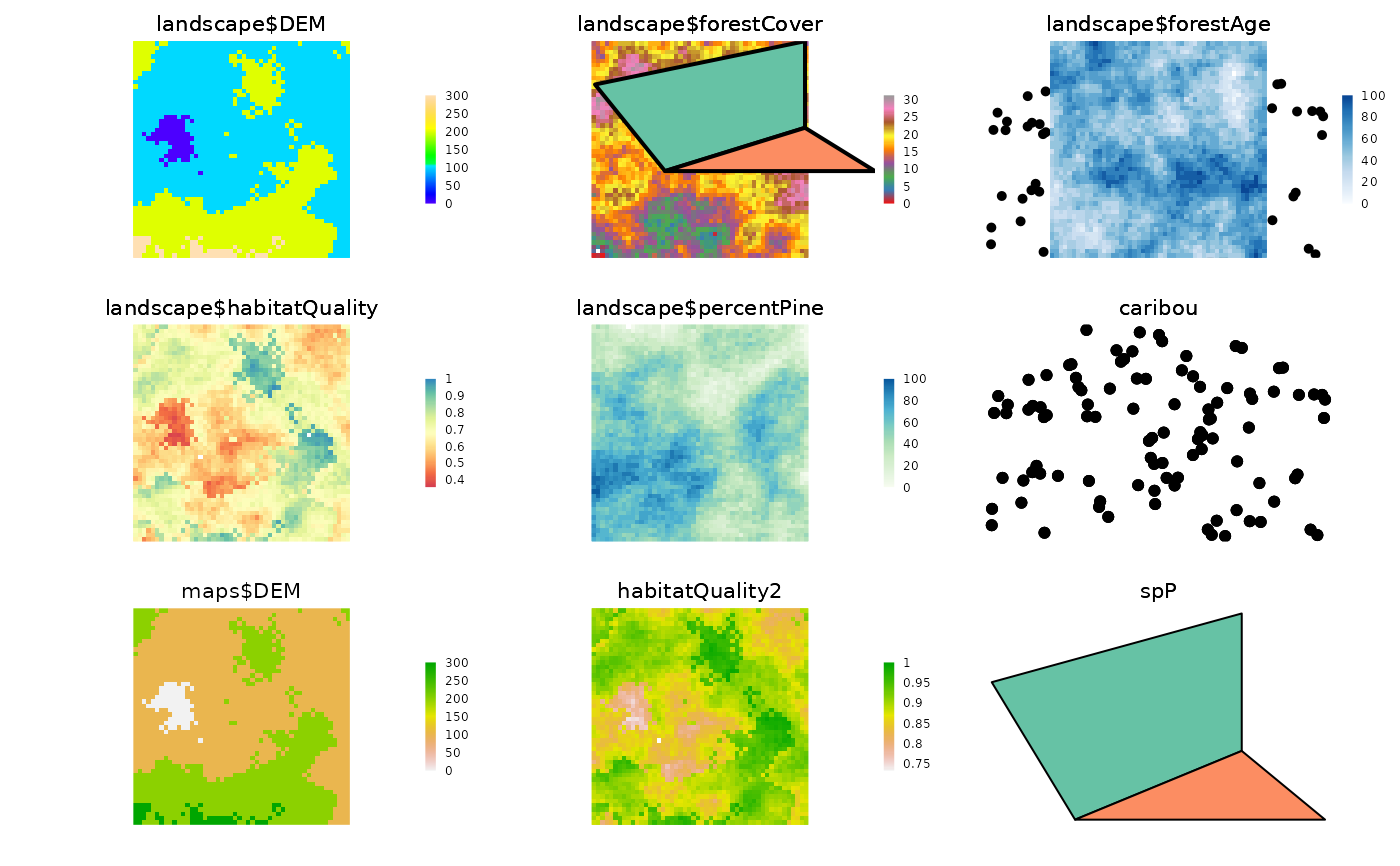# example base plot clearPlot()Plot(1:10, 1:10, addTo = "test", new = TRUE) # if there is no "test" then it will make itPlot(4, 5, pch = 22, col = "blue", addTo = "test")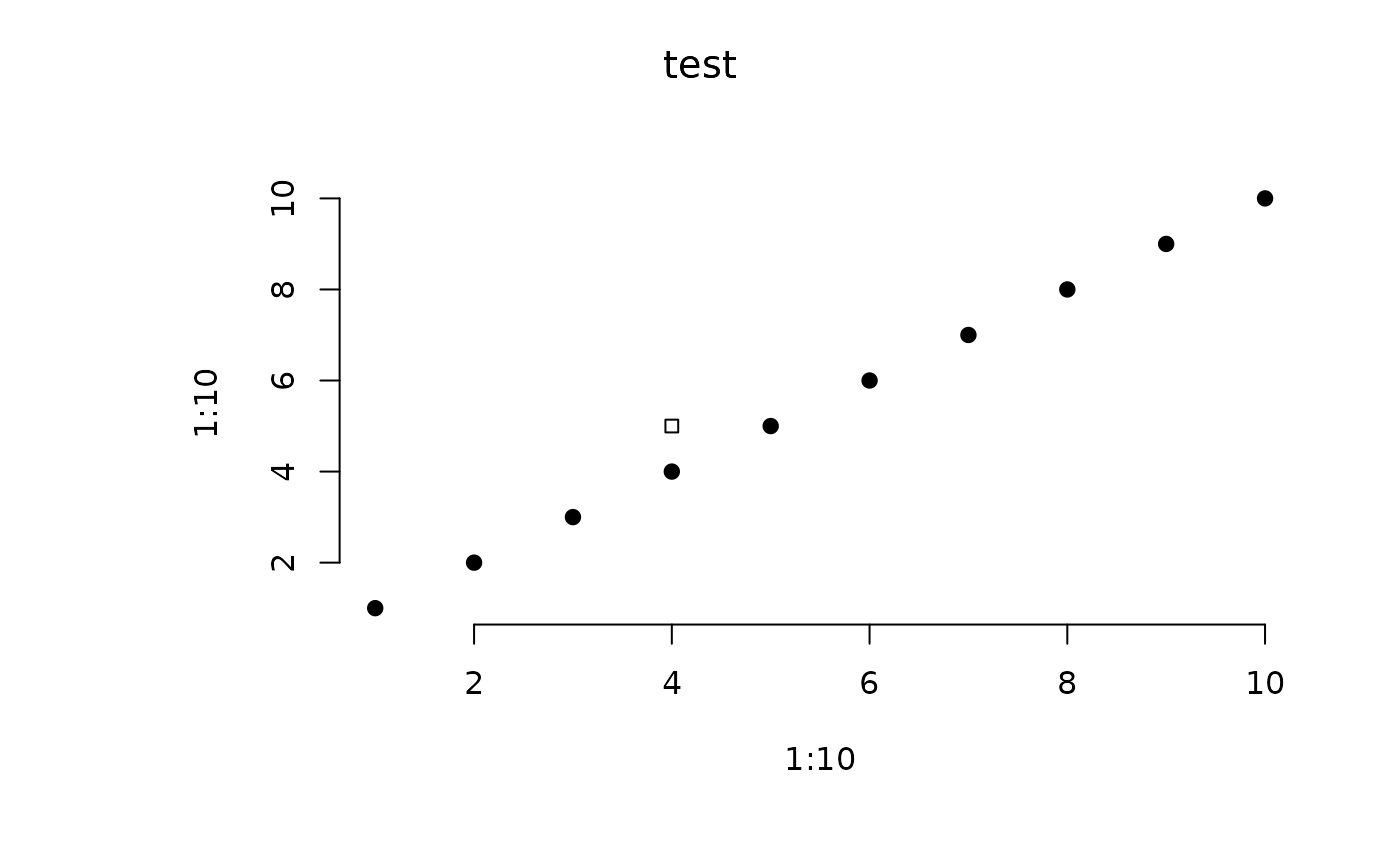obj1 <- rnorm(1e2) Plot(obj1, axes = "L")# Can plot named lists of objects (but not base objects yet) ras1 <- ras2 <- ras a <- list() for (i in 1:2) { a[[paste0("ras", i)]] <- get(paste0("ras", i)) } a$spP <- spP
clearPlot()Plot(a)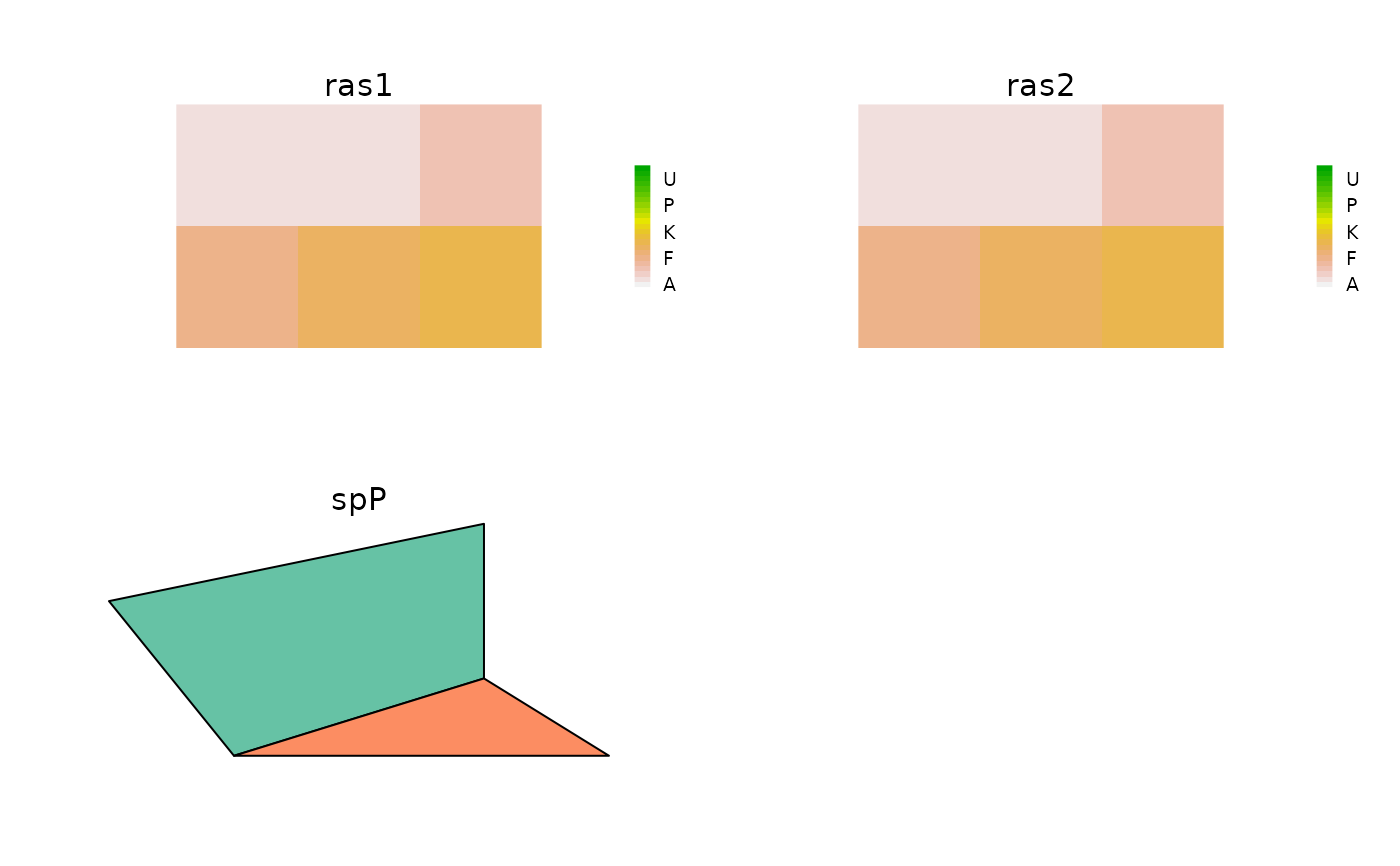clearPlot()